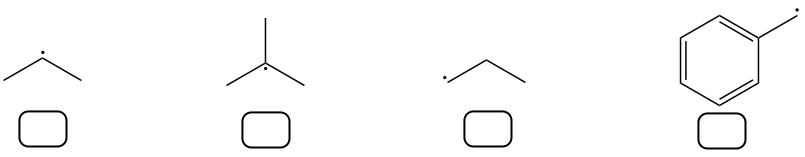Problem: Rank the following radicals in order of stability (1 = most stable, 4 = least stable).

FREE Expert Solution
82% (247 ratings)
Problem Details

Rank the following radicals in order of stability (1 = most stable, 4 = least stable).What scientific concept do you need to know in order to solve this problem?

Our tutors have indicated that to solve this problem you will need to apply the Radical Stability concept. You can view video lessons to learn Radical Stability Or if you need more Radical Stability practice, you can also practice Radical Stability practice problems .

What is the difficulty of this problem?

Our tutors rated the difficulty of Rank the following radicals in order of stability (1 = most ... as medium difficulty.

How long does this problem take to solve?

Our expert Organic tutor, Chris took 1 minute to solve this problem. You can follow their steps in the video explanation above.

What professor is this problem relevant for?

Based on our data, we think this problem is relevant for Professor Kachur's class at ROWAN.